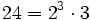# Symmetric group on finite set

## Definition

A symmetric group on finite set or symmetric group of finite degree is a symmetric group on a finite set.

See symmetric group for more general information about symmetric groups.

## Particular cases

### Small finite values

Since alternating groups are simple for degree at least five, all symmetric groups of degree at least five are not solvable. Also, all symmetric groups of degree greater than two are centerless, and among them, the one of degree six is the only one that is not complete.

Cardinality of set Common name for symmetric group of that degree Order with prime factorization Comments
0 Trivial group 1 Trivial
1 Trivial group 1 Trivial
2 Cyclic group:Z2 2 group of prime order. In particular, abelian
3 Symmetric group:S3$6 = 2 \cdot 3$ supersolvable but not nilpotent. Also, complete
4 Symmetric group:S4$24 = 2^3 \cdot 3$ solvable but not supersolvable or nilpotent. Also, complete
5 Symmetric group:S5$120 = 2^3 \cdot 3 \cdot 5$ not solvable. Has simple non-abelian subgroup of index two. Also, complete
6 Symmetric group:S6$720 = 2^4 \cdot 3^2 \cdot 5$ not solvable, and not complete.
7 Symmetric group:S7$5040 = 2^4 \cdot 3^2 \cdot 5 \cdot 7$ complete, not solvable, has simple non-abelian subgroup of index two.
8 Symmetric group:S8$40320 = 2^7 \cdot 3^2 \cdot 5 \cdot 7$ complete, not solvable, has simple non-abelian subgroup of index two.

## Group properties

Below we discuss properties satisfied for the symmetric group of degree$n$.

Property Satisfied? Explanation Comment
abelian group No for$n \ge 3$
nilpotent group No for$n \ge 3$
solvable group No for$n \ge 5$
complete group Yes for$n \ne 2,6$ symmetric groups are complete
centerless group Yes for$n \ne 2$ symmetric groups are centerless
ambivalent group Yes symmetric groups are ambivalent
strongly rational group Yes symmetric groups are strongly rational